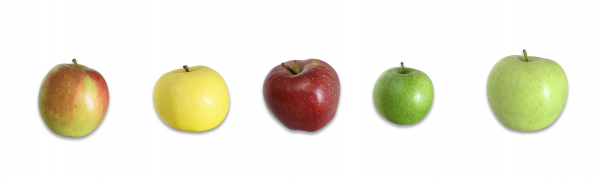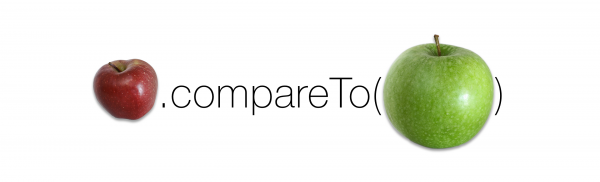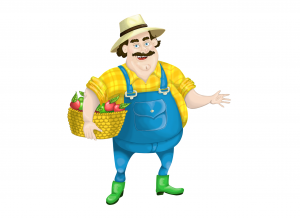Over a million developers have joined DZone.{{announcement.body}}
{{announcement.title}}

Java Comparable Interface in Five Minutes

DZone 's Guide to

Java Comparable Interface in Five Minutes

Part of Marcus Biel's Java 8 course focused on clean code principles, here is a tutorial on what the Java Comparable Interface is and how it's used.

· Java Zone ·
Free Resource

Comment (7)

Save
{{ articles.views | formatCount}} Views

In this short article, we will have a quick look at the Java Comparable Interface.

What is the Comparable Interface Used For?

How should we compare and sort things? Now that might seem like a weird question, but I want you to really think about it. Let’s say we have a set of apples:Example 1

How do we want to sort them? Do we want to sort them by weight? If so, are we sorting them from lightest to heaviest or heaviest to lightest? When we are sorting them, we need to repeatedly compare two apples' weights until all the apples are in the correct order. Is Apple 1 heavier than Apple 2? Is it heavier than Apple 3? We need to keep doing that until the list is sorted. The comparable interface helps us accomplish this goal. Comparable can’t sort the objects on its own, but the interface defines a method int compareTo(T).

How compareTo() Works

Let’s begin by utilizing the compareTo() method to see which apples are heavier.Example 2

The compareTo() method works by returning an int value that is either positive, negative, or zero. It compares the object by making the call to the object that is the argument. A negative number means that the object making the call is “less” than the argument. If we were comparing the apples by size, the above call would return a negative number, say -400, because the red apple is smaller than the green apple. If the two apples were of equal weight, the call would return 0. If the red apple was heavier, compareTo() would return a positive number, say 68.

The Flexibility of compareTo()

If we called the compareTo() method above repeatedly, we could sort our apples by size, which is great, but that’s not the end of the story. What if we want to sort apples by color? Or weight? We could do that too. The key is that our client, let’s call him Fatty Farmer, (see Example 3), needs to precisely define how the apples need to be sorted before we can start development.Example 3

He can do this by answering these two questions:

1. How does he want the apples to be sorted? What is the characteristic he would like us to compare?
2. What does ‘less than’, ‘equal to’, and ‘greater than’ mean in that context?

It’s also possible to use multiple characteristics, as we’ll see a little bit later.

Example 1: Sorting Apples By Weight

For our first example, we’re going to sort our apples by weight. It only requires one line of code.

``Collections.sort(apples);``

Example 4

The above line of code can do all the sorting for us, as long as we’ve defined how to sort the apples in advance (That’s where we’ll need more than one line).

Let’s begin by writing the apple class.

``````public class Apple implements Comparable<Apple> {
private String variety;
private Color color;
private int weight;

@Override
public int compareTo(Apple other) {
if (this.weight < other.weight) {
return -1;
}
if (this.weight == other.weight) {
return 0;
}
return 1;
}
}``````

Example 5

This is our first version of class Apple. Since we are using the compareTo method and sorting the apples, I implemented the Comparable interface. In this first version, we’re comparing objects by their weight. In our compareTo() method we write an if condition that says if the apple’s weight is less than the other apple, return a negative number, to keep it simple, we’ll say -1. Remember, this means that this apple is lighter than Apple ‘other’. In our second if statement, we say that if the apples are of equal weight, return a 0. Now if this apple isn’t lighter, and it isn’t the same weight, then it must be greater than the other apple. In this case we return a positive number, say, 1.

Example 2: Sorting Apples By Multiple Characteristics

As I mentioned before, we can also utilize compareTo() to compare multiple characteristics. Let’s say we want to first sort apples by variety, but if two apples are of the same variety, we should sort them by color. Finally, if both of these characteristics are the same, we will sort by weight. While we could do this by hand, in full, like I did in the last example, we can actually do this in a much cleaner fashion. Generally, it is better to reuse existing code than to write our own. We can use the compareTo methods in the Integer, String, and enum classes to compare our values. Since we aren’t using Integer objects, rather we are using ints we have to use a static helper method from the Integer wrapper class to compare the two values.

``````public class Apple implements Comparable {
private String variety;
private Color color;
private int weight;

@Override
public int compareTo(Apple other) {
int result = this.variety.compareTo(other.variety);
if (result != 0) {
return result;
}
if (result == 0) {
result = this.color.compareTo(other.color);
}
if (result != 0) {
return result;
}
if (result == 0) {
result = Integer.compare(this.weight, other.weight);
}
return result;
}
}``````

Example 6

In Example 6, we compare the first quality of the apples that our client prioritized, their variety. If the result of that compareTo() call is non-zero, we return the value. Otherwise we make another call until we get a non-zero value, or we’ve compared all three characteristics. While this code works, it isn’t the most efficient or clean solution. In Example 3, we refactor our code to make it even simpler.

``````@Override
public int compareTo(Apple other) {
int result = this.variety.compareTo(other.variety);
if (result == 0) {
result = this.color.compareTo(other.color);
}
if (result == 0) {
result = Integer.compare(this.weight, other.weight);
}
return result;
}``````

Example 7

As you can see, this greatly shortens our code and allows us to make each comparison in only one line. If the result of a compareTo() call is zero, we just move on to the next “round” of comparisons within the same if statement. This, by the way, is a good example of what you do as a Clean Coder. Usually, you don’t instantly write Clean Code; you start with a rough idea, make it work, and then continuously improve it until you’ve made it as clean as you can.

Comparable, hashCode, and Equals

You may notice that the compareTo() looks a little bit like the hashCode() and equals() methods. There is one important difference, however. For hashCode() and equals(), the order in which you compare individual attributes does not influence the value returned, however in compareTo() the order of the objects is defined by the order in which you compare the objects.

Conclusion

To conclude I just want to underscore how important the Comparable interface is. It is used in both the java.util.Arrays and the java.util.Collections utility classes to sort elements and search for elements within sorted collections. With collections like TreeSet and Tree Map, it’s even easier — they automatically sort their elements which have to implement the Comparable interface.

Topics:
java 8 ,interface ,beginner guide ,video

Comment (7)

Save
{{ articles.views | formatCount}} Views

Published at DZone with permission of

Opinions expressed by DZone contributors are their own.